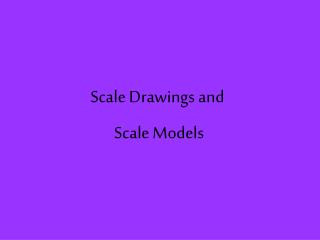DownloadDownload PresentationScale Drawings and Scale Models

# Scale Drawings and Scale Models

Télécharger la présentation## Scale Drawings and Scale Models

- - - - - - - - - - - - - - - - - - - - - - - - - - - E N D - - - - - - - - - - - - - - - - - - - - - - - - - - -
##### Presentation Transcript

1. Scale Drawings and Scale Models

2. Vocabulary scale drawing: proportional two-dimensional drawing of an object (smaller or larger) scale model: proportional three-dimensional model of an object (smaller or larger) scale factor: a ratio of the model/drawing’s dimensions to the actual object’s dimensions in simplest form scale: ratio between two sets of measurements

3. Examples of Scale Drawings blueprints maps

4. Examples of Scale Models toy car model of a house toy airplane

5. Finding Scale Factor model’s dimensions actual object’s dimensions

6. Caution!!! A scale factor is ALWAYS the ratio of the model’s dimensions to the actual object’s dimensions.

7. Caution!! It’s alright to have a scale factor greater than 1. If the scale factor is greater than 1, this means that the new figure is bigger than the original (think about cells). If the scale factor is less than 1, then the new figure is smaller than the original (think about model airplanes).

8. Finding a Scale Factor You can use the lengths or heights to find the scale factor. MODEL LENGTH 20 AIRPLANE LENGTH 1,800 THEN SIMPLIFY! ÷ 20 20 1 = 1,800 ÷ 20 90 SCALE FACTOR!

9. Finding a Scale Factor You can use the lengths or heights to find the scale factor. 8.4 MODEL HEIGHT AIRPLANE HEIGHT 756 THEN SIMPLIFY! 8.4 ÷ 8.4 1 = 756 ÷ 8.4 90 SCALE FACTOR!

10. Finding a Scale Factor MODEL HEIGHT 10 35 GREAT DANE HEIGHT THEN SIMPLIFY! 2 10 ÷ 5 = 35 ÷ 5 7 SCALE FACTOR!

11. Using Scale On a road map with a scale of 1 cm: 120 mi., the distance between two cities measures 3.5 cm. What is the actual distance between these two cities? MODEL 1 cm 3.5 cm = 120 mi x mi ACTUAL 420 ÷ 1 = 420 The actual distance between these two cities is 420 miles.

12. Using Scale Jeffery is making a model of a car. He uses the scale 1 in:12 in. If the length of the actual car is 132 in., what is the length of his model car? MODEL 1 in x in = 12 in 132 in ACTUAL 132 ÷ 12 = 11 The length of his model car is 11 in.

13. Using Scale Factor Kelly enlarged her favorite photograph into a poster. The poster is 22 inches by 38 inches. The scale factor is 6/1. Find the size of the original photograph. Round to the nearest tenth. 22 inches x inches x inches 38 inches

14. Using Scale Factor Kelly enlarged her favorite photograph into a poster. The poster is 22 inches by 38 inches. The scale factor is 6/1. Find the size of the original photograph. Round to the nearest tenth. Let’s find length first! 6 in MODEL 38 in = ACTUAL x in 1 in 38 ÷ 6 = 6.3

15. Using Scale Factor Kelly enlarged her favorite photograph into a poster. The poster is 22 inches by 38 inches. The scale factor is 6/1. Find the size of the original photograph. Round to the nearest tenth. 22 inches x inches 6.3 inches 38 inches

16. Using Scale Factor Kelly enlarged her favorite photograph into a poster. The poster is 22 inches by 38 inches. The scale factor is 6/1. Find the size of the original photograph. Round to the nearest tenth. Now let’s find the width! 6 in MODEL 22 in = x in ACTUAL 1 in 6 = 22 ÷ 3.6

17. Using Scale Factor Kelly enlarged her favorite photograph into a poster. The poster is 22 inches by 38 inches. The scale factor is 6/1. Find the size of the original photograph. Round to the nearest tenth. 22 inches 3.6 inches 6.3 inches 38 inches

18. Now we know... The original size of the photograph is 3.6 inches by 6.3 inches. In this case, the scale factor was larger than one. This means, that the model is larger than the original.

19. Try This: On a scale drawing of a horse, the scale is 1 mm: 10 mm. If the real horse is 1,500 mm tall, how tall is the horse on the scale drawing?